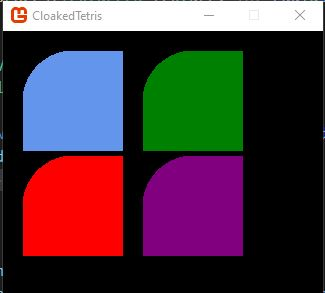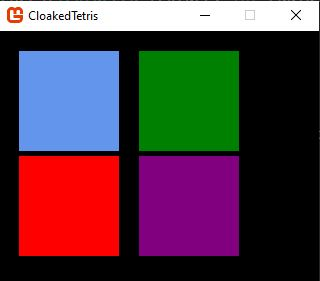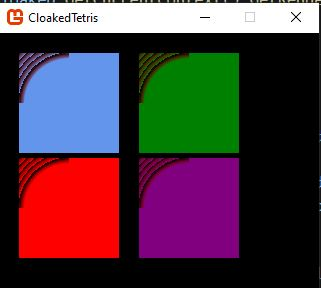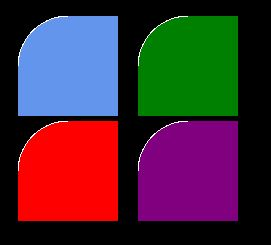# How to make part of texture transparent with SetData?

Hi there. I am fairly novice with Monogame but have done enough searching on my own without finding answers to warrant making a post here, I think.

I am working on a UI system that creates colored textures from scratch by calling SetData on Texture2D.

I want to be able to make rounded corners dynamically based on a radius value. This is what I have so far (it is incomplete, and only changes the top left corner so far):

``````        public static void ApplyCorners(Texture2D texture, int radius)
{

for (int row = 0; row < radius; row++)
{
for (int column = 0; column < radius; column++)
{
Vector2 current = new Vector2(row, column);
float distance = Vector2.Distance(current, origin);
float margin = radius - distance;
Color color = data[row * radius + column];
if (margin < 0)
{
if (margin < -1) data[row * radius + column] = Color.Transparent;
else data[row * radius + column] = new Color(color.R, color.G, color.B, (byte)(byte.MaxValue * ((1 + margin) / 5)));
}
else if (margin < 5)
{
data[row * radius + column] = new Color(color.R, color.G, color.B, (byte)(byte.MaxValue * (margin / 5)));
}
}
}
``````

And that gets me this result:As you can see my algorithm for rounding the corners is correct but regions where I would expect partial transparency are still fully opaque.

Changing
`if (margin < -1) data[row * radius + column] = Color.Transparent;`
to
`if (margin < -1) data[row * radius + column] = new Color(color.R, color.G, color.B, (byte)(byte.MaxValue * ((1 + margin) / 5)));` gets me this:Which isn’t rendering any transparency in the corner as I would expect it to.

My spriteBatch code is as follows (these two methods are called in succession):

``````    private void DrawToRenderTarget(SpriteBatch spriteBatch)
{
GraphicsDeviceManager.GraphicsDevice.SetRenderTarget(RenderTarget);
spriteBatch.Begin(SpriteSortMode.Immediate, BlendState.AlphaBlend);
RenderablesInScope.ForEach((r) => r.Draw(spriteBatch));
spriteBatch.End();
GraphicsDeviceManager.GraphicsDevice.SetRenderTarget(null);
}

private void DrawRenderTarget(SpriteBatch spriteBatch)
{
spriteBatch.Begin(SpriteSortMode.Immediate, BlendState.AlphaBlend);
GraphicsDeviceManager.GraphicsDevice.Clear(Color.Red);
spriteBatch.Draw(RenderTarget, ViewPort, Camera.ViewRectangle, Color.White);
spriteBatch.End();
}
``````

and changing BlendState to `NonPremultiplied` gives me this result (with the previous change in place):This leads me to believe that something about the way I am creating my texture (I am not loading it from the contend pipeline but creating it manually) is not pre multiplying alpha and causing the alpha not to work in AlphaBlend mode.

Am I correct? And what can I do to solve this?

Maybe check what values are actually going into the alpha when you write this, and compare to the data that’s expected to go in. Just looking at it, it looks like you’re using the byte overload, but that repeating pattern on the part that’s supposed to be transparent has be suspicious that you’re overflowing the value.

So yea, check what you’re actually writing to the colour value for those pixels. Is it what you expect?

I had that suspicion too but was able to rule it out. None of the alpha values are overloaded, and I wouldn’t expect them to be since I am multiplying the max byte value by a fractional number. It wouldn’t explain the lack of transparency when using AlphaBlend either.

That may be your intent, but I suspect that’s not actually what’s happening.

When margin is greater than 4, the resulting alpha value will exceed byte.MaxValue.

Likewise, when margin is greater than 5, you will also have an alpha value that exceeds byte.MaxValue.

If the result clamps to 255, you’d end up with a solid colour, like what you see in your second image. If the result wraps around, you’d end up with a pattern such as what you see in your third image.

It might be worth another look, maybe even setting a conditional breakpoint on margin for when its value is greater than 4 or 5 and observe what gets put into data. What sometimes also helps when debugging is to assign the new colour to a temporary variable before putting it into data, so that you can more easily find the value in the debugger.

Anyway, give this a try and let me know. If the values look good internally, I might try making a small demo application with your code to see what I can find.

I have already done the things you suggest (breakpoints, output all the A values, etc). That code has gone through several iterations and I probably should have posted this version, which has the same problem and is not as confusing (sorry):

``````                        if (margin >= -1) data[row * radius + column] = Color.Transparent;
else data[row * radius + column] = new Color(color.R, color.G, color.B, (byte)(((int)byte.MaxValue) * (1 + margin)));
``````

It’s important to note that in the above code margin will always be a negative number, and when I do that multiplication it is always between -1 and 0.

Here is the full method in case you want to test it out:

``````        public static void ApplyCorners(Texture2D texture, int radius)
{

for (int row = 0; row < radius; row++)
{
for (int column = 0; column < radius; column++)
{
Vector2 current = new Vector2(row, column);
float distance = Vector2.Distance(current, origin);
float margin = radius - distance;
Color color = data[row * radius + column];
if (margin < 0)
{
if (margin <= -1) data[row * radius + column] = Color.Transparent;
else data[row * radius + column] = new Color(color.R, color.G, color.B, (byte)(((int)byte.MaxValue) * (1 + margin)));

if (margin > -1) Console.WriteLine(\$"[{(byte)(byte.MaxValue * (1 + margin))}]");
}
else if (margin < 5)
{
data[row * radius + column] = new Color(color.R, color.G, color.B, (byte)(byte.MaxValue * (margin / 5)));
}
}
}
}
``````

Here is how I am generating the texture to begin with:

``````Texture2D texture = new Texture2D(Cloaked.GraphicsDeviceManager.GraphicsDevice, 100, 100);
Color[] data = new Color[100 * 100];
for (int i = 0; i < 100 * 100; i++) data[i] = _color;
texture.SetData(data);
Utilities.ApplyCorners(texture, 50);``````

The texture gets sent to the GPU unaltered. If you want to use premultiplied alpha, you have to do that yourself. Premultiplied alpha just means that the RGB values are multiplied by the alpha value.

Or you use NonPremultiplied, like you did. It seems to be working alright. There are just some extra waves in your screenshot because the margin value got too far into the negative.

I went through your algorithm and it’s actually a bit confusing. What’s happening is that your calculations are yielding a higher alpha value farther out than you want. You’re right in that it’s not exceeding the max value of a byte, I’m not sure what you were doing to get that image above that looks like wrap around, but in this iteration there was nice blending except at the edges, where the pixels were brighter than usual.

I tried to understand what you were doing, but I’m having trouble figuring out your intent. So I figured instead I’d just provide an example that’s working probably closer to how you want it to be, then you can compare it with what you’re doing.

Anyway, I think this is probably the effect you’re looking for…

``````		private void ApplyCorners(Texture2D texture, int radius)
{
float blendRange = 1f;

for (int row = 0; row < radius; row++)
{
for (int column = 0; column < radius; column++)
{
Vector2 current = new Vector2(row, column);
float distance = Vector2.Distance(current, origin);
Color color = data[row * radius + column];

if (distance > radius + blendRange)
{
color.A = 0;
}
{
float amt = (distance - radius) / blendRange;
color.A = (byte)(byte.MaxValue * (1 - amt));
}

data[row * radius + column] = color;
}
}
}
``````

You can change blendRange to tweak the effect.

I don’t think we are on the same page, honestly. Consider this code:

``````                if (margin < 0)
{
if (margin <= -1)
{
data[row * radius + column] = Color.Transparent;
}
else if (margin > -5)
{
Color c = new Color(color.R, color.G, color.B, (byte)(((int)byte.MaxValue) * (1 + margin / 5)));
data[row * radius + column] = c;
}
}
``````

The code that applies the modified A value is in a condition that makes it so it will always give me valid numbers. `1 + margin / 5` will always be less that 1 because margin is always `margin < 0` and `margin > -5`.

And yet this is the result:To see what should be transparent in that image, I can change `data[row * radius + column] = c;` to `data[row * radius + column] = Color.White;` to get this:@Trinith I think I figured it out. My algorithm wasn’t right but it wasn’t the margin value that was wrong.

This logic:

``````                    if (margin <= 0)
{
data[row * radius + column] = Color.Transparent;
}
else if (margin < 3)
{
data[row * radius + column] = new Color(color.R, color.G, color.B, (byte)(byte.MaxValue * (margin / 3)));
}
``````

Gives me a nice smoot corner:Anyway thank you for taking the time to trouble shoot this with me!

1 Like

Just saw your reply. I got my algo working but I will take a look at yours too. I think I was just having a hard time getting the logic right, as you said.

Thank you!

1 Like

One issue though is that it doesn’t apply alpha in AlphaBlend mode, which I am not sure if that’s okay or not.

I considered the code from your first post with the wave pattern screenshot:

``````if (margin < -1) data[row * radius + column] = new Color(color.R, color.G, color.B, (byte)(byte.MaxValue * ((1 + margin) / 5)));
``````

What you ended up with is pretty much the same as what I had